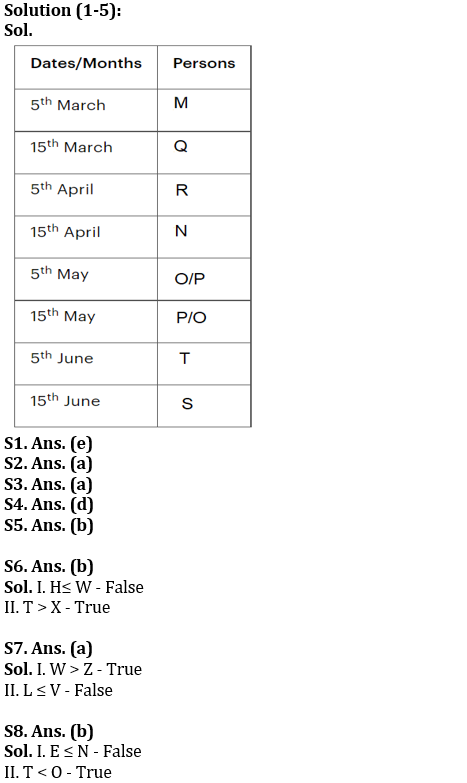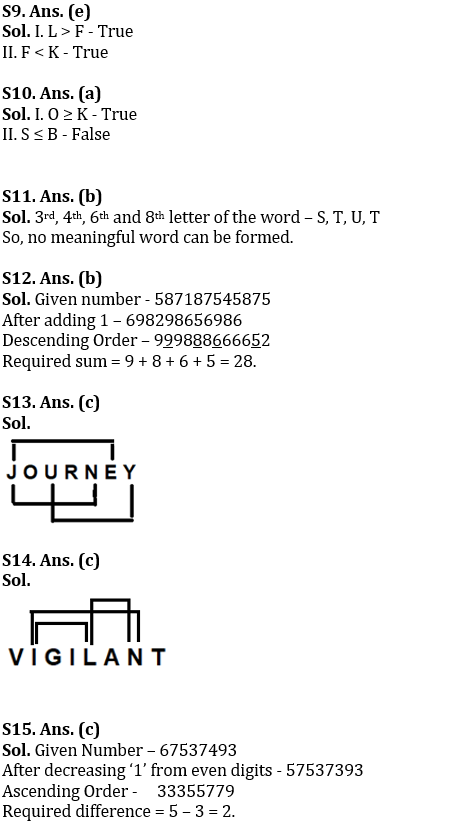Latest Banking jobs   »

# Reasoning Ability Quiz For IBPS RRB PO Prelims 2022- 1st July

Directions (1-5): Answer the questions based on the information given below.
Eight persons M, N, O, P, Q, R, S and T have examinations on two different i.e., 5th or 15th of four different months (March, April, May, and June) of the same year but not necessarily in the same order.
M has exam on a prime numbered date. Three persons have exams between T and R. O and P has exam in the same month. Two persons have exams between M and N. Neither N nor R has exam in March and May. S and T have exam in the same month but after N.

Q1. Who among the following person has exam on 5th May?
(a) Q
(b) M
(c) O
(d) P
(e) Can’t be determined

Q2. How many persons have exam between M and R?
(a) One
(b) Two
(c) Three
(d) Four
(e) More than four

Q3. Q has the exam on which of the following date?
(a) 15th March
(b) 5th May
(c) 15th April
(d)15th June
(e) None of these

Q4. If P has exam immediately before T, then how many persons have exams before O?
(a) One
(b) Two
(c) Three
(d) Four
(e) More than four

Q5. Who among the following person has exam immediately after R?
(a) Q
(b) N
(c) O
(d) P
(e) None of these

Directions (6-10): In the following questions assuming the given statement to be true, find which of the conclusion(s) among given conclusions is/are definitely true and then give your answers accordingly.

Q6. Statements: T>W >U; X < U > M > K; H<K
Conclusions: I. H≤ W
II. T > X
(a) Only I is true
(b) Only II is true
(c) Either I or II is true
(d) Neither I nor II is true
(e) Both I and II are true

Q7. Statements: V>W ≥ I > Q; L=F < Y < Q; L > Z
Conclusions: I. W > Z
II. L ≤ V
(a) Only I is true
(b) Only II is true
(c) Either I or II is true
(d) Neither I nor II is true
(e) Both I and II are true

Q8. Statements: E ≤ T ≤ H = J, N= O > C ≥ R, J < N
Conclusions: I. E ≤ N
II. T < O
(a) Only I is true
(b) Only II is true
(c) Either I or II is true
(d) Neither I nor II is true
(e) Both I and II are true

Q9. Statements: I = N < K, T > F ≤ I, L = H ≥ T
Conclusions: I. L > F
II. F < K
(a) Only I is true
(b) Only II is true
(c) Either I or II is true
(d) Neither I nor II is true
(e) Both I and II are true

Q10. Statements: B = K > E, R = D < O ≥ B, G > S ≥ R
Conclusions: I. O ≥ K
II. S ≤ B
(a) Only I is true
(b) Only II is true
(c) Either I or II is true
(d) Neither I nor II is true
(e) Both I and II are true

Q11. If it is possible to make a meaningful word from 3rd, 4th, 6th and 8th letter of the word ‘DESTRUCTION, from the left end, by using the letters only once, then what will be the 3rd letter of that meaningful word so formed? If no such meaningful word can be formed, then mark the answer as ‘Y’, and if more than one meaningful word can be formed then mark the answer as ‘X’.
(a) X
(b) Y
(c) S
(d) T
(e) U

Q12. If we add “1” to all the digits of the number ‘587187545875’, and then, arrange the new digits in descending order from the left end. Now find, what will be the sum of 2nd, 5th, 7th, and 11th digits from the left end after the rearrangement?
(a) 30
(b) 28
(c) 27
(d) 24
(e) None of these

Q13. How many such pair of letters are there in the word ‘JOURNEY‘ each of which have as many letters between them (in forward and backward direction) as in the English alphabet?
(a) None
(b) Two
(c) Three
(d) Four
(e) None of these

Q14. How many such pair of letters are there in the word ‘VIGILANT‘ each of which have as many letters between them (in forward and backward direction) as in the English alphabet?
(a) None
(b) Two
(c) Three
(d) Four
(e) None of these

Q15. If all the even digits of the number ‘67537493’ are decreased by ‘1’ and then the new digits are arranged in ascending order from left to right, then what will be the difference of third digit from left end and fifth digit from the right end?
(a) 3
(b) 4
(c) 2
(d) 5
(e) None of these

Solutions#### Congratulations!Incorrect details? Fill the form again here

•Reasoning Ability Quiz For IBPS RRB PO C...
•Reasoning Ability Quiz For IBPS Clerk Pr...
•Reasoning Ability Quiz For IBPS RRB PO C...
•Reasoning Ability Quiz For IBPS RRB PO C...
•Reasoning Ability Quiz For IBPS RRB PO C...
•Reasoning Ability Quiz For IBPS Clerk Pr...